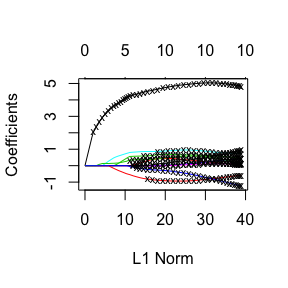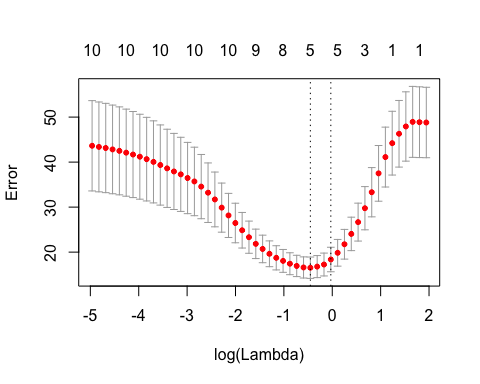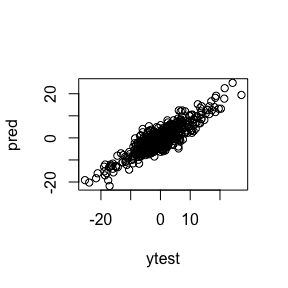Introduction to pliable

Introduction

pliable is a package that fits the pliable lasso, a new method for obtaining sparse models for supervised learning problems. The pliable lasso takes as input the usual feature matrix X and response y , but also a matrix of modifying variables Z. These variables may be continuous or categorical. The pliable lasso model is a generalization of the lasso in which the coefficients multiplying the features X are allowed to vary as a function of the modifying variables Z.

We introduce some notation that we will use throughout this vignette. Let there be $$n$$ observations, each with feature vector $$x_i \in \mathbb{R}^p$$ and response $$y_i$$. Let $$X \in \mathbb{R}^{n \times p}$$ denote the overall feature matrix, and let $$y \in \mathbb{R}^n$$ denote the vector of responses. Finally, let $$Z \in \mathbb{R}^{n \times nz}$$ denote the matrix of modifying variables.

pliable fits the model

$\hat y = \beta_0+ Z\theta_0+ \sum_j X_j(\beta_j + Z\theta_j)$

where $$X_j$$ is the $$j$$th column of $$X$$. Here each $$\beta_j$$ is a scalar and each $$\theta_j$$ is a vector of length $$nz$$. The model is fit with penalties that encourage both $$\beta=(\beta_1, \ldots \beta_p)$$ and each $$\theta_j$$ to be sparse, and also a hierarchy constraint that allows $$\theta_j$$ to be non-zero only if $$\beta_j$$ is non zero. Note that each $$\theta_j$$ represents an interaction between $$X_j$$ and all of $$Z$$. Details of the optimization may be found in the paper by Tibshirani and Friedman arXiv.

pliable uses cyclical coordinate descent, which successively optimizes the objective function over each parameter with all other parameters fixed, cycling repeatedly until convergence. The interaction terms $$\theta_j$$ are estimated by proximal gradient descent.

The package also includes methods for prediction and plotting, and a function which performs $$k$$-fold cross-validation.

Installation

We begin by installing pliable from CRAN. Type the following command in R console:

install.packages("pliable")

This command downloads the R package and installs it to the default directories. Users may change add a repos option to the function call to specify which repository to download from, depending on their locations and preferences.

Alternatively, users can download the package source at CRAN and type Unix commands to install it to the desired location.

Quick Start

The purpose of this section is to give users a general sense of the package. We will briefly go over the main functions of the package, as well as some key options. More details are given in later sections.

First, we load the pliable package:

library(pliable)
#> Loaded glmnet 2.0-16

Let’s generate some data:

set.seed(944)
n = 50; p = 10 ;nz=5
x = matrix(rnorm(n*p), n, p)
mx=colMeans(x)
sx=sqrt(apply(x,2,var))
x=scale(x,mx,sx)
z =matrix(rnorm(n*nz),n,nz)
mz=colMeans(z)
sz=sqrt(apply(z,2,var))
z=scale(z,mz,sz)
y =4*x[,1] +5*x[,1]*z[,3]+ 3*rnorm(n)

We recommend that the columns of both $$X$$ and $$Z$$ be standardized, before running pliable, as we have done above.

We fit the model using the most basic call to pliable:

fit <- pliable(x,z,y)

The function pliable returns a pliable object. We can examine the results


fit
#>
#> Call:  pliable(x = x, z = z, y = y)
#>
#>         Lambda      %Dev Num of main effects Num with ints Tot num of ints
#>  [1,] 6.986000 0.7196000                   0             0               0
#>  [2,] 6.067000 0.6642000                   1             0               0
#>  [3,] 5.269000 0.6156000                   1             0               0
#>  [4,] 4.576000 0.5789000                   1             0               0
#>  [5,] 3.975000 0.5511000                   1             0               0
#>  [6,] 3.452000 0.5231000                   1             1               1
#>  [7,] 2.998000 0.4606000                   1             1               1
#>  [8,] 2.604000 0.3984000                   2             1               1
#>  [9,] 2.262000 0.3431000                   2             1               1
#> [10,] 1.964000 0.2916000                   3             1               1
#> [11,] 1.706000 0.2488000                   4             1               1
#> [12,] 1.482000 0.2132000                   4             1               1
#> [13,] 1.287000 0.1853000                   4             1               1
#> [14,] 1.118000 0.1623000                   5             1               1
#> [15,] 0.970600 0.1443000                   5             2               2
#> [16,] 0.843000 0.1296000                   5             2               2
#> [17,] 0.732200 0.1184000                   5             2               2
#> [18,] 0.635900 0.1098000                   5             2               2
#> [19,] 0.552300 0.1033000                   5             3               3
#> [20,] 0.479700 0.0976900                   6             3               3
#> [21,] 0.416600 0.0925400                   6             6               6
#> [22,] 0.361800 0.0832000                   8            13              13
#> [23,] 0.314200 0.0745800                   8            15              15
#> [24,] 0.272900 0.0671500                   8            15              15
#> [25,] 0.237000 0.0604400                   8            19              19
#> [26,] 0.205900 0.0538800                   9            24              24
#> [27,] 0.178800 0.0465200                   9            25              25
#> [28,] 0.155300 0.0402800                   9            26              26
#> [29,] 0.134900 0.0344500                  10            31              31
#> [30,] 0.117100 0.0294100                  10            30              30
#> [31,] 0.101700 0.0251100                  10            31              31
#> [32,] 0.088360 0.0213100                  10            33              33
#> [33,] 0.076740 0.0178700                  10            34              34
#> [34,] 0.066650 0.0148800                  10            35              35
#> [35,] 0.057890 0.0123600                  10            35              35
#> [36,] 0.050270 0.0101400                  10            36              36
#> [37,] 0.043660 0.0083050                  10            36              36
#> [38,] 0.037920 0.0068150                  10            36              36
#> [39,] 0.032940 0.0055310                  10            39              39
#> [40,] 0.028610 0.0044760                  10            40              40
#> [41,] 0.024840 0.0035860                  10            41              41
#> [42,] 0.021580 0.0028630                  10            41              41
#> [43,] 0.018740 0.0022970                  10            41              41
#> [44,] 0.016280 0.0018560                  10            42              42
#> [45,] 0.014140 0.0014870                  10            42              42
#> [46,] 0.012280 0.0012160                  10            42              42
#> [47,] 0.010660 0.0009830                  10            43              43
#> [48,] 0.009261 0.0008054                  10            43              43
#> [49,] 0.008043 0.0006685                  10            45              45
#> [50,] 0.006986 0.0005506                  10            45              45

For each model in the solution path indexed by $$\lambda$$, the table shows the % Deviance explained, number of main effects (non-zero $$\hat\beta_j$$s) , number of main effects with interactions (number of $$\hat\theta_j$$ vectors that have at least one zero component) and the number of non-zero interactions (non-zero $$\hat\theta_{jk}$$ values.) We can plot the path of coefficients

plot(fit)And we can make predictions using a pliable object by calling the predict method. Each column gives the predictions for one value of lambda. Here we choose the 20th value:

# get predictions for 20th model
predict(fit, x[1:5, ],z[1:5,])[,20]
#>    3.903689 -14.024664  15.182350   2.066809  -2.925222

Cross-validation (CV)

We can perform $$k$$-fold cross-validation (CV) with cv.pliable. It does 10-fold cross-validation by default:

cvfit <- cv.pliable(fit,x, z, y)

We can change the number of folds using the nfolds option:

cvfit <- cv.pliable(fit, x, z,y , nfolds = 5)

If we want to specify which observation belongs to which fold, we can do that by specifying the foldid option, which is a vector of length $$n$$, with the $$i$$th element being the fold number for observation $$i$$.

foldid <- sample(rep(seq(5), length = n))
cvfit <- cv.pliable(fit, x,z,y, foldid = foldid)

A cv.pliable call returns a cv.pliable object. We can plot this object to get the CV curve with error bars (one standard error in each direction). The left vertical dotted line represents lambda.min, the lambda value which attains minimum CV error, while the right vertical dotted line represents lambda.1se, the largest lambda value with CV error within one standard error of the minimum CV error.

plot(cvfit)The two special lambda values can be extracted directly from the cv.pliable object as well:

cvfit$lambda.min #>  0.6359002 cvfit$lambda.1se
#>  0.9706495

Predictions can be made from the fitted cv.pliable object. By default, predictions are given for lambda being equal to lambda.1se. To get predictions are lambda.min, set s = "lambda.min".

set.seed(44)
ntest=500
xtest = matrix(rnorm(ntest*p),ntest,p)
xtest=scale(xtest,mx,sx)
ztest =matrix(rnorm(ntest*nz),ntest,nz)
ztest=scale(ztest,mz,sz)
ytest = 4*xtest[,1] +5*xtest[,1]*ztest[,3]+ 3*rnorm(ntest)
pred= predict(fit,xtest,ztest,lambda=cvfit$lambda.min) plot(ytest,pred)Categorical Z If $$Z$$ is categorical, you much first convert it to set of dummy variables (one-hot encoding’’). Suppose that that you have two $$Z$$ variables, a categorical one $$Z1= (3,1,4,2,2,1,3)$$ and a quantitative variable $$Z2$$. Then you would convert $$Z1$$ to dummy variables, using say $$D=model.matrix(~Z)$$ and then define $$Z$$ as $$Z <- cbind(D,Z2)$$ Z not observed in test set Here is an example where Z is not observed in the test set, but predicted from a supervised learning algorithm  n = 50; p = 10 ;nz=5 x = matrix(rnorm(n*p), n, p) mx=colMeans(x) sx=sqrt(apply(x,2,var)) x=scale(x,mx,sx) z =matrix(rnorm(n*nz),n,nz) mz=colMeans(z) sz=sqrt(apply(z,2,var)) z=scale(z,mz,sz) y =4*x[,1] +5*x[,1]*z[,3]+ 3*rnorm(n) fit = pliable(x,z,y) # predict z from x; here we use glmnet, but any other supervised method can be used zfit=cv.glmnet(x,z,family="mgaussian") # Predict using the fitted model ntest=500 xtest =matrix(rnorm(ntest*nz),ntest,p) xtest=scale(xtest,mx,sx) ztest =predict(zfit,xtest,s=cvfit$lambda.min)[,,1]
ytest = 4*xtest[,1] +5*xtest[,1]*ztest[,3]+ 3*rnorm(ntest)

pred= predict(fit,xtest,ztest)

Other options

Here are some other options that one may specify for the pliable and cv.pliable functions:

• w: The user can pass a vector of length $$n$$ representing observation weights. The squared residual of the observations are weighted according to this vector. By default, this is set to 1 for all observations.

• family: The default value for the family option of the pliable and cv.pliable functions is gaussian. Use this default when y is a quantitative variable (i.e. takes values along the real number line). For binary prediction, use family = binomial. In this setting, the response y should be a numeric vector containing just 0s and 1s.

• lambda: The lambda sequence at which the model fit will be computed. This is typically not provided by the user: the program can construct the sequence on its own. When automatically generated, the lambda sequence is determined by lambda.max (internally computed) and lambda.min.ratio. (lambda.min.ratio is the ratio of smallest value of the generated lambda sequence, say lambda.min, to lambda.max.) The program generates nlam values (default is 50) linear on the log scale from lambda.max down to lambda.min.

• standardize: If set to TRUE, the columns of the feature matrix x are scaled to have unit variance before the algorithm is run.

For more information, type ?pliable or ?cv.pliable.